# Discrete Mathematics - why and when?

### "How the discrete precedes the continues."

Michiel Doorman & Heleen Verhage
Freudenthal Institute, University of Utrecht, The Netherlands

## 1 Introduction

Discrete mathematics is a rapidly growing branch in mathematics. It has many applications in business and is strongly related to computer science. Discrete mathematics is a very broad area in mathematics. The name is introduced this century for a number of topics that deal with finite states, e.g. coding problems, path finding problems, recursion, production graphs, and population-prediction matrices. The problems turn to such areas as finding structures in situations, turning a situation into a model, and learning how to assess this model. Some rather traditional topics of discrete mathematics which are or were in the past in many countries part of the mathematics curriculum are sequences and combinatorics.

There is a tendency all over the world to pay more attention to discrete mathematics in education. Reasons for this can be found both outside and inside education. The main reason from outside education is the increasing importance of discrete mathematics in society, which is a result of the recent developments in computational sciences.

From an educational point of view there are also several arguments to give discrete mathematics a more prominent place in the curriculum. In the first place discrete mathematics in itself is worth to be taught, because it can contribute to general goals of mathematics education, like problem solving, recognising structure, reasoning, learning to generalise and mathematical modelling. In the second place there are didactical arguments to teach discrete mathematics.

At the moment in the USA most changes in the teaching and learning of mathematics at primary and secondary school are based on the framework that is given by the NCTM Curriculum and Evaluation Standards for School Mathematics. The standard for discrete mathematics includes the following sentences (NCTM 1989, p.176)):

"Whereas the physical or material world is most often modelled by continuous mathematics, that is, the calculus and prerequisite ideas from algebra, geometry, and trigonometry, the non-material world of information processing requires the use of discrete (discontinuous) mathematics. Computer technology, too wields an ever-increasing influence on how mathematics is created and used. (...) In light of these facts it is crucial that all students have experiences with the concepts and methods of discrete mathematics."

Some of the recommendations formulated in these Standards are (NCTM Yearbook 1991, Preface):

"Discrete mathematics:

• promotes the making of mathematical connections
• provides a setting for problem solving with real world applications
• capitalizes on technological settings
• fosters critical thinking and mathematical reasoning."

Another argument for discrete mathematics is that the subject is valuable in education for didactical reasons. In our opinion a discrete approach can contribute to the learning and teaching of rather classic learning areas like algebra and calculus. Discrete problems are quite often rather easy to understand, which doesn't mean that these problems are easy to solve (two very famous counter examples are the four-colour problem and Fermat's last theorem!). Is this equivalent with the fact that the principle of counting is easier than the principle of measurement, where very soon limit processes and infinity play a role?

Both adults and (even very young) children are easily fascinated by patterns, which often have a numerical basis, or can be analysed by counting something. This makes looking at patterns or regularities a good starting point for the learning of mathematics. We hold a plea to make use of these phenomena and start with discrete activities. In our opinion discrete mathematics can become an important chain in a long learning sequence: from arithmetic to calculus, with the algebra in between.

The aim of this paper is to give an impression of discrete mathematics and its possible place in such a long learning sequence in secondary education, by means of several examples. But before we come to that, some words are spent to sketch the philosophy of realistic mathematics education, which is the background of the mainstream in mathematics education in the Netherlands at the moment.

##### Realistic mathematics education

The role of context problems used to be limited to the applications that would be addressed at the end of a learning sequence - as a kind of add on. Nowadays, context problems have a more central role. They are endorsed, because of today's emphasis on the usefulness of what is learned, and because of their presumed motivational power. Context problems play an even more encompassing role in the Dutch approach that is known as realistic mathematics education (RME). In RME the role of context problems is not limited to the end of a sequence. Instead, they play a role from the start onwards. The point of departure is that contextual problems that are experientially real for the students, can function as anchoring points for the reinvention of mathematics by the students themselves.

In reform mathematics in general, and in the case in RME especially, the way the role of context problems is perceived relates to a vision on mathematics education. In contrast with the traditional view in which teaching mathematics was seen as a process according to a pre-designed hierarchy, mathematics teaching in the reformed view is seen as helping the students to develop their own mathematics. It is in this light that context problems are interwoven in the RME approach.

##### Contents of this paper

This paper contains mainly examples. These examples show both the realistic approach and the way how discrete mathematics can contribute to a long learning sequence related to numbers, tables, formulas, (linear) algebra and calculus.

Paragraph 2 is devoted to some problems that can be done at junior secondary level. Paragraph 3 concentrates on a discrete approach to calculus. Paragraph 4 gives some examples on graph theory and matrices, while paragraph 5 is devoted to discrete dynamic modeling, a very new topic in the Dutch curriculum at upper secondary level. In paragraph 6 some conclusions are formulated.

## 2 Tables, graphs and formulas

All over the world at junior secondary education teachers and learners struggle with the teaching and learning of algebra. In RME tables, graphs and formulas are used at all levels and seen as powerful tools for the learning of algebra.

So in junior secondary education students become familiar with tables, graphs and formulas to represent a situation. Tables, graphs and formulas are seen as mathematical tools that can be of help in the process of modeling a context situation. Depending on the situation, the students choose the appropriate tool, and are supposed to jump in a flexible way from one tool to another. This process can be visualised by the following graph: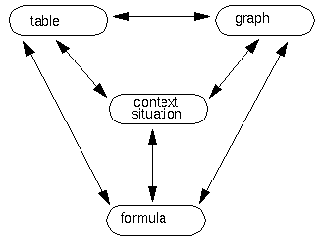One of the goals of mathematics education is that students learn how to construct formulas by themselves. Of course this is not an easy goal to reach, it is part of a long term learning process. By means of carefully chosen, realistic problem situations their informal knowledge is used, and the students are motivated to move towards more formal strategies. In this way algebraic language and concepts are developed, and time is taken for this. A very natural way to let the students make formulas, can be found in all kinds of discrete situations.

A possible way to structure a mathematics learning line is by increasing mathematical complexity: first linear functions, solving linear equations, then quadratic functions and quadratic equations, and so on. Much time is spend on manipulations with variables and expressions, which are often more complicated than necessary to solve problems about linear and quadratic functions. Many techniques are practised rather isolated.

In the RME approach, the importance of the study of relationships between variables remains, but with different emphasis. First of all, the relationships are presented in realistic problems and are not only linear and quadratic relationships, also exponential and more complex relationships are explored. The concepts of all different relationships are developed parallel to each other together with strategies that can be applied in more than one specific situation. This approach is a shift from purely disjunct vertical mathematization to horizontal mathematization. It can be seen as a change of the time factor (Abels 1996):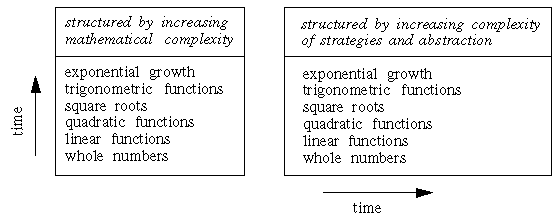The global idea is to start with activities where students have to look for regularities and patterns, and write down their results in a table. By doing this, they make use of common sense and knowledge from primary education. The next step is that they try to generalise what they have found and make a formula to describe the pattern. At this stage a shift from arithmetic to mathematics takes place for most students. The following three examples give an impression of this process.

##### Example 1 - Counting rods

The following example is taken from the unit Building Formulas: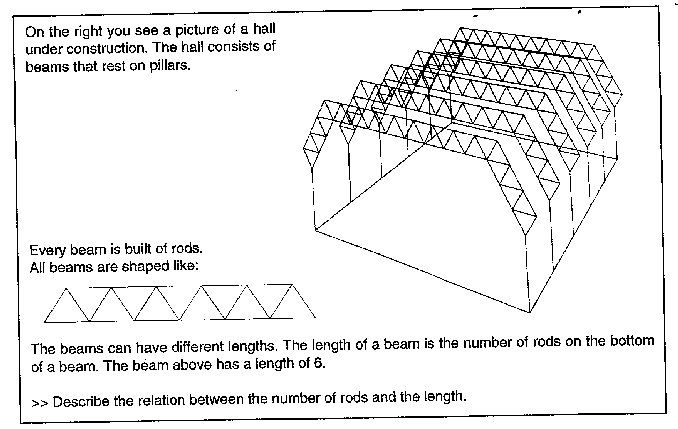Classroom experiments learned that different students come up with different formulas for the number of rods, like:

L x 3 + (L - 1)

L + L x 2 + (L - 1)

4 L - 1

These different solutions can be compared and discussed in class. To begin with, this can be done in relation to the context: the pattern of the beam can be seen in different ways, which leads to different formulas. In a rather natural way the relation with formal algebraic rules comes up, like adding terms and working with parentheses.

Building formulas is important, because students learn to look at patterns in a systematic way, and to find structure and use mathematics as a tool to describe structures. While building formulas students become familiar with thinking in terms like `current' and `next'. Notions that play a role when it comes to describe how a variable changes over time.

##### Example 2 - Counting oranges

The following example is based on a problem taken from a chapter on formulas in the Dutch textbook Moderne Wiskunde:

A greengrocer fills a pyramid shaped glass container with layers of oranges, as follows: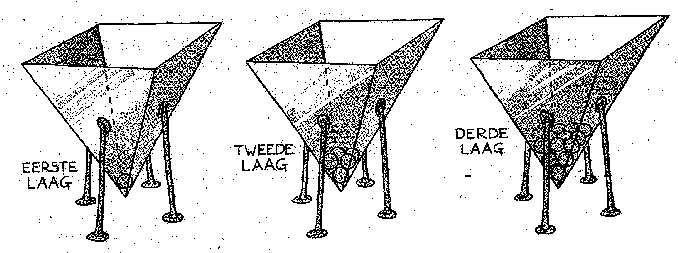The number of oranges in each layer can be written down in a table:

 number of layer 1 2 3 4 5 6 ... number of oranges in layer 1 4 9 16 25 36 ...

The first row in the table gives the number of the layer in the glass container, the second row gives the number of oranges in each layer. The greengrocer uses the formula `number of layer times number of layer' to calculate the number of oranges in a arbitrary layer.

At a rather basic level it is possible to ask questions like: `How many layers can you make if there are 150 oranges available?'

Tables like these can play an important role in long learning sequences, because the table can be extended with a third row, meant for the differences of the values in the second row. This new row is the so called `difference sequence'.

 difference row 3 5 7 9 11 ...

A direct formula to describe the difference sequence appears to be 2n + 1.

This process can be continued, and it is also possible to make a row with the differences of the difference sequence. The result is the so called `sequence of second differences', which appears to be a constant sequence.

 row of second differences 2 2 2 2 ...

This can also be done the other way around: given a difference sequence, the problem is to find the original sequence. So it appears that the difference sequence gives information on the original sequence.

##### Example 3 - The growth of a sunflower

Looking at differences can also be done in graphs.

This can be illustrated by the following graph which describes the growth of a sunflower: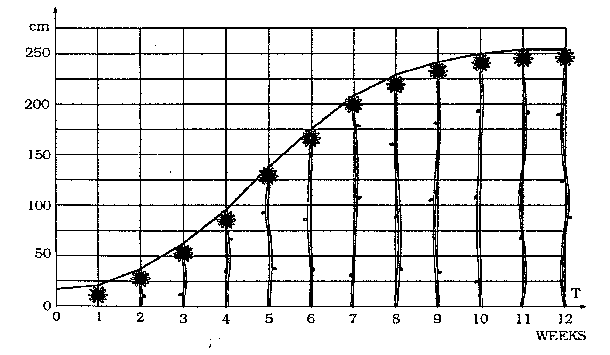Looking at differences is also possible in graphs, like this graph of the growth of a sunflower. In this graph three different types of growth can be recognised: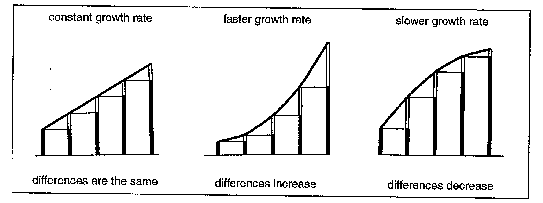The differences are represented by the white sticks. These sticks can be drawn in so-called `difference diagrams':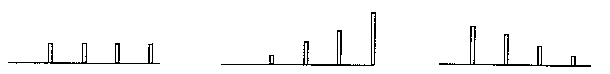For every graph a difference diagram can be made. And, the other way around, difference diagrams can be used to get information on the original graph.

##### Looking at discrete differences

The above examples show that tables, graphs and formulas are very powerful mathematical tools. The examples 2 and 3 show also how these tools can be extended by looking at differences.

The three tools: tables, graphs and formulas can all be extended in this way, as follows:

* tables < -- > difference tables

* (global) graphs < -- > (global) differences diagrams

* formulas < -- > difference formulas.

The didactical idea behind this focus on (discrete) differences is to let it be an informal and intuitive preparation for calculus, one of the main topics of senior secondary education in most countries. Our experience is, that a basis for the main notions and concepts of calculus can already be laid at junior secondary level, by means of this discrete approach. In the Netherlands we felt the need to look for a different approach to calculus, because the more traditional one appeared not to be very successful.

## 3 A different approach to calculus

Before we sketch an approach to calculus from discrete mathematics we try to indicate the problems of a traditional start of calculus education which is inspired by David Tall (1991).

##### A traditional start of calculus education

Mathematicians tend to make a typical error when they design an instructional sequence for calculus: try to simplify a complex mathematical topic by breaking it up in smaller parts, that can be ordered in a sequence that is logical from a mathematical point of view. From the view point of the expert, the individual parts together constitute a coherent whole. But the student interprets every part as an isolated subject, like a piece of a jigsaw without knowing the complete picture. The result may be that the students construct an image of each individual piece, without ever succeeding in bringing all pieces together in one whole.

As an example, to be able to understand the derivative f'(x), one has to have the concept of a limit at one's disposal. For, one has to take the limit of the difference quotient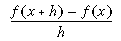where h tends to zero. Thus the concept of a limit has to precede the derivative. Further, one might decide that it is easier to take the limit in the case where x is fixed. The next step then would be to let x vary, to introduce the idea of a derivative in this manner. Following this mathematically sensible line of reasoning, the following sequence will emerge:

#### introduction of the concept of limit

1. introduction of the limit for a fixed x = a:
2.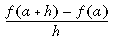as h tends to zero which will be called f'(a)

1. exchanging a for x, and varying x to create the derivative f'(x).

For the student, however, the first step mysteriously appears out of the blue for no reason, with all cognitive problems. The next big problem is in the shift from 2 to 3, since taking a limit in one point is an activity that substantially differs from perceiving f'(x) as a function of which its values describe the gradient of a graph of f(x).

An alternative start that avoids a formal introduction of the limit concept in the beginning is from discrete mathematics. This is not so strange when we look at some aspects of the history of calculus. First, we give a short impression of Galileo's attribution to Calculus. In a following example we show how this history can be used for a textbook for students.

##### Galileo and free fall

Galileo conjectured that an object in a free fall moves with a constant acceleration, and that you can conclude from this that the falling distance is proportional to the square of time. This last conclusion was based on a reasoning with graphs from Oresme in the 14th century. Oresme approximated the distance traversed from the time-velocity graph. Let the velocity be fixed for a certain short time-interval, the distance traversed in that time-interval equals the length of the interval times the velocity. This value can be represented by the area of the rectangle with the time-interval as base and the velocity as height. When you repeat this for all the time-intervals in the time-velocity graph you get a grasp of the distance traversed: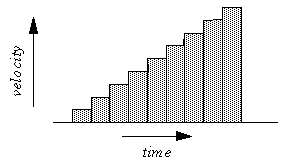The diagonal top-line of the graph, results when you choose the time-intervals very small. He noticed that it is a straight line and that the sum of the areas of all the rectangles is an approximation of the area of the triangle between the top-line and the horizontal axis.

When an object moves in this way with a velocity from 0 to v in a time interval t, then the distance traversed is equal to the area of the triangle: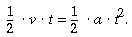Galileo repeated the construction in the seventeenth century, and wanted to apply this formula on free fall. He used the ratio between the distance traversed in equal time intervals. This ratio is 1:3 when you divide time in two intervals: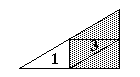It is 1:3:5:7 when you divide time in 4 intervals, and it stays a ratio determined by a sequence of odd numbers. Galileo knew that such sequences added up to a square and with this property he tested the formula. He designed a slide with nails at a side. The distances between the nails were in a ratio with the odd numbers. A rolling ball should need the same time to pass each following nail. It was difficult for Galileo to measure the time-intervals. It is possible that he used his heart beat to test the equal time-intervals, others think he might have sung a song to generate a constant rhythm.

An instrument from the 19th century that could be used for the experiment of Galileï: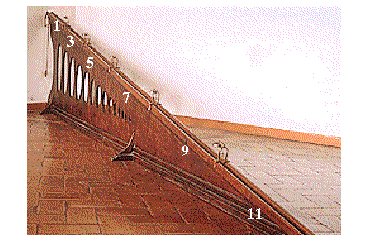Galileo found with his experiments that the falling distance increases linear each time-interval and from this result he concluded that the relation between distance and time must be quadratic.

This short historical sketch shows four important aspects in the development of calculus:

#### No formal limit-concept

1. The importance of discrete analysis
2. The importance of graphing
3. The relation between the development of mathematics and problems from real-life phenomena

This history teaches us that the use of graphs played an important role. Global interpretations of areas under graphs and approximations of these areas with rectangles were the first instruments. Also important to note is that the area of these rectangles had a meaning in the context. It was a discrete analysis of a continuous process. In addition can be noted that first graphs were used in relation with the area under the graph, and centuries later tangents of graphs were determined in relation to derived functions. At last we want to remark that familiar series and sequences can be used to determine discrete approximations of areas or differences, it might be helpful to know that the difference of two following squares is an odd number (see the example of the oranges).

##### From the discrete to the continuous

It seems that the context of velocity-time and distance-time formula's and graphs has a good perspective to be used for the development of calculus. Students are familiar with informal or global reasoning problems about velocity-time and distance-time graphs.

Velocity-time graphs can be used in the way like Oresme and Galileo used them to introduce the concept of the area under a graph and to introduce ways to calculate them. The following examples are taken from the unit Som&Verschil, which is the introduction on differential calculus (the exercise below is based on a text by Polya, 1963).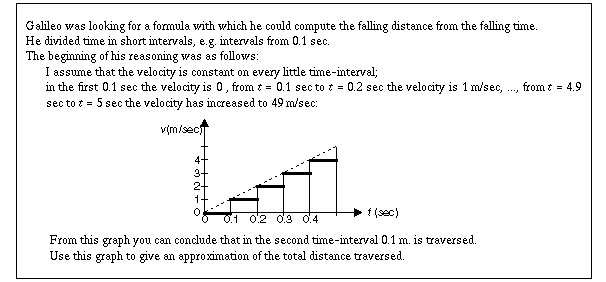Further more, the relation between sums and differences are developed. For the example above this can be illustrated with the following graph of y = x2.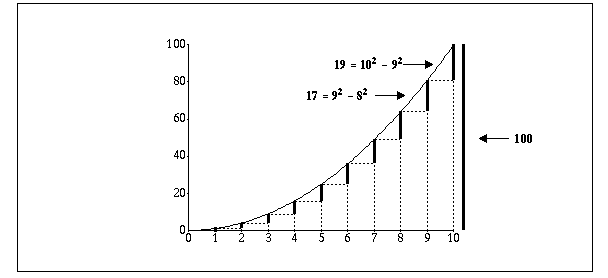Knowledge of series and sequences can be used to understand that difference-formulas of quadratic formulas always are linear.

Distance-time graphs can also be used for the development of a differential quotient as a way to determine the average speed on a small time-interval. When that is understood students can be asked to find a way, or an algorithm, to determine the instantaneous velocity at a certain moment from the distance-time graph.

These properties are visualised with graphs. Students use graphic calculators when dealing with these investigations: locally straightness is a way to determine the velocity at a certain moment.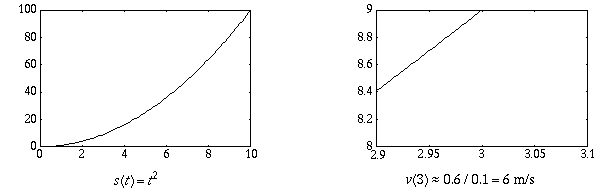In this calculus sequence, the central model is that of a discrete function, in combination with it's representation as a discrete graph. Discrete functions lay the basis for both integration and differentiation via sum functions and difference functions. Moreover, the basis for the main theorem of calculus is laid by investigating the relation between sum functions and difference functions.

## 4 Graphs and Matrices

At senior secondary education there are different streams of mathematics education in the Netherlands. The so called mathematics A is meant for students who have in mind to study economics or social sciences, while mathematics B prepares for studies like technical sciences, mathematics, physics, etc. One of the goals of mathematics A is the building of mathematical models. In the eighties the curriculum of the mathematics A course was developed, and one of the topics was Graphs and Matrices.

Graphs are an important model for many problems in discrete mathematics. They are helpful for structuring a problem. With a graph you can extract and display the information you want to investigate. Another model that is used very often is the matrix. One of the features of matrices is that you can make computations with them. When a discrete process is represented by a matrix, in some cases the powers of the matrix can give extra information about this process. Both the graph and the matrix are seen very often in everyday life, e.g. railway-maps and distance-tables.

A graph can be represented as a matrix, and very often you can draw a graph to display the information from a given matrix. In this way you can create translation activities.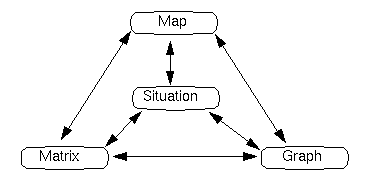The names for graphs and matrices depend upon the mathematical context in which they are used, such as connectivity graphs, production graphs, and population prediction graphs. In each case, the numbers within the matrices may take on different meanings. The different meanings of numbers in different situations, and in otherwise identical looking matrices, make this subject a challenging one in mathematics. Students must reflect continuously on what they are doing, both from a mathematical point of view and a contextual point of view.

##### Multiplication with matrices

Edges in graphs can have 'static' meanings (e.g. distances between vertices) and 'dynamic' meanings (e.g. the probability to come from one vertex to the other). In the latter case the graph-model can be used for computations. Typical problems here deal with population prediction models and with Markov processes. Students discover with these problems what kind of results you get when you make many computations with these models (e.g. population prediction graphs give always exponential or cyclic growth).

##### Example 2 - Detergents

The following example is a characteristic problem of the applied Mathematics A course in the Netherlands: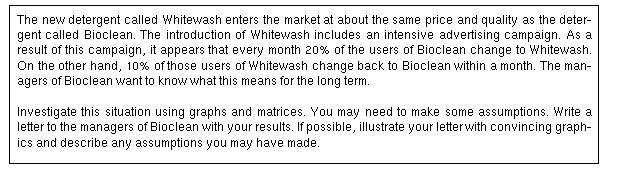This situation can be modelled by the graph: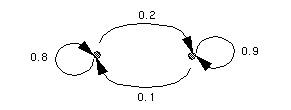with the related matrix model: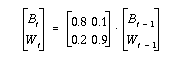which can be analysed in a numerical way by means of a computer program. Below you see a line graph of the computed results of a similar problem against time: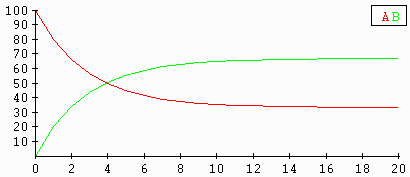## 5 Discrete dynamic modelling

A very new topic in mathematics A is `Discrete Dynamic Modeling'. This is not even introduced yet at a nationwide scale, in is still in the stage of experiments at the moment.

The mathematical content of this new module is:

• recursive processes
• dynamic demand/supply models (a context taken from economics)
• first order linear difference equations
• different graphical representations of dynamic processes.
• difference equations related to matrices.

The following diagram shows how the domain Discrete Dynamic Modelling is related to other domains in the curriculum: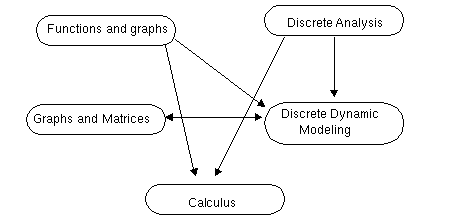This new domain is related to several other domains in the curriculum and gives a good opportunity to practice the building of mathematical models. Students start with numerical computations to investigate situations. They find different types of growth and in some cases the existence of equilibrium values. Another way to investigate the situations is to enter the formulas in a graphic calculator and interpret the graphs. At last, the most abstract way is to solve the equations algebraically. But this is not always possible. We will illustrate these three approaches with an example about forestry and a return to the example on detergents in the preceding paragraph.

##### Example - Forestry

An introductory problem that can be explored numerically with the graphic calculator: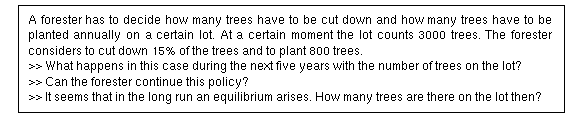For calculations like:

new number = 0.85 * old number + 800

the ans -button of the graphic calculator is very useful: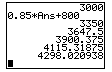Iteration leads to the assumption that the size of the forest changes in the direction of an equilibrium. An `ordinary' graph with the number of trees U(n) against time is: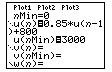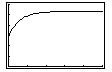A second type of graph is the so called web, with the old value on the horizontal axis and the new value on the vertical axis. By a repetitive process of transferring new to old (by means of the graph of y = x) the equilibrium is found as the intersection of the graphs of:y = x and y = 0.85x + 800: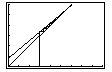This example shows how the three tools tables, graphs and formulas are used again, with the graphic calculator as a valuable help.

##### Example - Detergents again

An interesting aspect of difference equations is, that there appears to be a relation between matrices and systems of linear difference equations. This relation can be illustrated by means of the detergent example presented before. The matrix model presented there can also be written as a system of difference equations:

B t = 0.8 B t-1 + 0.1 W t-1
W t = 0.2 B t-1 + 0.9 W t-1

Because B t + W t = 100 (the total of market shares is constant and equal to 100%) this can be rewritten to

B t = 0,7 B t-1 + 10

which is a straight forward first order linear difference equation . By applying some knowledge of geometric series it appears that the solution of this difference equation for B t is

B t = 33,3 + 66,7 0,7 t

So the theory of difference equations can also be applied on matrix models. In the past at secondary schools matrix models were only analysed in a numerical way, but now for the 2x2 models the students have also an analytical tool to their disposal as well.

## 6 In conclusion

Here we want to cite Susan Picker (1996): "An important aspect of discrete mathematics is, that it enables students to see mathematics more accurately, as having many problems which are still unsolved. With only a small amount of technical background students can understand enough about a class of problems such as graph colouring to understand that no algorithm exists which will always guarantee the most efficient colouring. It means that students can stand at the cutting edge of mathematical knowledge with the real possibility of making a contribution; something they could not have previously believed.

There are a number of other student beliefs about mathematics that discrete mathematics seriously challenges. Such widely held beliefs as mathematics problems can only have one right answer, that doing maths means memorizing formulas and giving them back in the right order at the right time, that most math problems can be solved within minutes, and that mathematics can only be created by geniuses, are disproved in a discrete mathematics class where there may be many answers to a problem, there may be no formula to follow, students may work on a problem over several days, and where students may see something that the teacher has never seen that way before."

The use of discrete mathematics for calculus education makes it possible to give students time to understand concepts like rate of change. The algorithms to solve problems in discrete mathematics are very close to the problem situations. In this way you prevent that the algebraic techniques for differentiation and integration don't seem to have any relation with the concepts, and as a result of this, students forget this relation. The algebraic techniques become the main object of study and not the "real" problems of calculus.

At last, it appeared that in the historical development of mathematical concepts almost always the discrete preceded the continues, and probably we can learn from this for mathematics education.

## 7 References

Abels, M. et al. (1993) Moderne Wiskunde, vol. 1 mavo-havo-vwo. Wolters-Noordhoff, Groningen.

Abels, M. (1996). A shift in algebra, development and implementation: varia-abilities. Proceedings of Working Group 13 at ICME-8. Freudenthal Institute, Utrecht.

Doorman, M. (1997). Mathematical modeling: Using Graphs and Matrices. Learning in Motion Inc. Including software.

Kenney, M.J. and C.R. Hirsch (1991). Discrete Mathematics across the Curriculum, K-12. 1991 Yearbook. USA: National Council of Teachers of Mathematics.

Kindt, M. (1996) Som & Verschil, Afstand & Snelheid. Differentiaal- en Integraalrekening, deel 1. Freudenthal Institute, Utrecht.

Polya, G. (1963). Studies in mathematics, volume XI, Mathematical Methods in Science. Stanford: School Mathematics Group.

Picker, Susan H. (1996). Curriculum Reform In New York City High Schools: Discrete Mathematics. Proceedings of Working Group 13 at ICME-8. Freudenthal Institute, Utrecht.

Sandefur, J.T (1993). Discrete Dynamic Modeling. New York: Oxford University Press.

Reeuwijk, M. and M. Wijers (1997), `Students' construction of Formulas in Context'. In Mathematics Teaching in the Middle School, Vol. 2, No. 4.